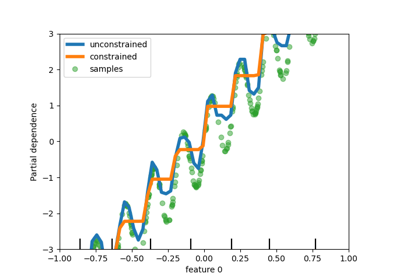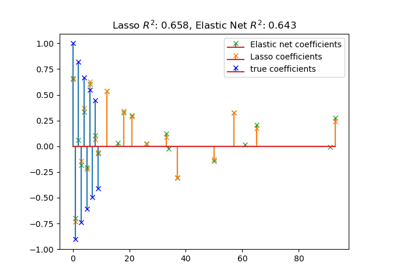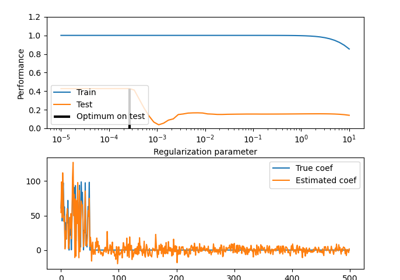# sklearn.linear_model.ElasticNet¶

class sklearn.linear_model.ElasticNet(alpha=1.0, *, l1_ratio=0.5, fit_intercept=True, normalize='deprecated', precompute=False, max_iter=1000, copy_X=True, tol=0.0001, warm_start=False, positive=False, random_state=None, selection='cyclic')[source]

Linear regression with combined L1 and L2 priors as regularizer.

Minimizes the objective function:

1 / (2 * n_samples) * ||y - Xw||^2_2
+ alpha * l1_ratio * ||w||_1
+ 0.5 * alpha * (1 - l1_ratio) * ||w||^2_2


If you are interested in controlling the L1 and L2 penalty separately, keep in mind that this is equivalent to:

a * ||w||_1 + 0.5 * b * ||w||_2^2


where:

alpha = a + b and l1_ratio = a / (a + b)


The parameter l1_ratio corresponds to alpha in the glmnet R package while alpha corresponds to the lambda parameter in glmnet. Specifically, l1_ratio = 1 is the lasso penalty. Currently, l1_ratio <= 0.01 is not reliable, unless you supply your own sequence of alpha.

Read more in the User Guide.

Parameters:
alphafloat, default=1.0

Constant that multiplies the penalty terms. Defaults to 1.0. See the notes for the exact mathematical meaning of this parameter. alpha = 0 is equivalent to an ordinary least square, solved by the LinearRegression object. For numerical reasons, using alpha = 0 with the Lasso object is not advised. Given this, you should use the LinearRegression object.

l1_ratiofloat, default=0.5

The ElasticNet mixing parameter, with 0 <= l1_ratio <= 1. For l1_ratio = 0 the penalty is an L2 penalty. For l1_ratio = 1 it is an L1 penalty. For 0 < l1_ratio < 1, the penalty is a combination of L1 and L2.

fit_interceptbool, default=True

Whether the intercept should be estimated or not. If False, the data is assumed to be already centered.

normalizebool, default=False

This parameter is ignored when fit_intercept is set to False. If True, the regressors X will be normalized before regression by subtracting the mean and dividing by the l2-norm. If you wish to standardize, please use StandardScaler before calling fit on an estimator with normalize=False.

Deprecated since version 1.0: normalize was deprecated in version 1.0 and will be removed in 1.2.

precomputebool or array-like of shape (n_features, n_features), default=False

Whether to use a precomputed Gram matrix to speed up calculations. The Gram matrix can also be passed as argument. For sparse input this option is always False to preserve sparsity.

max_iterint, default=1000

The maximum number of iterations.

copy_Xbool, default=True

If True, X will be copied; else, it may be overwritten.

tolfloat, default=1e-4

The tolerance for the optimization: if the updates are smaller than tol, the optimization code checks the dual gap for optimality and continues until it is smaller than tol, see Notes below.

warm_startbool, default=False

When set to True, reuse the solution of the previous call to fit as initialization, otherwise, just erase the previous solution. See the Glossary.

positivebool, default=False

When set to True, forces the coefficients to be positive.

random_stateint, RandomState instance, default=None

The seed of the pseudo random number generator that selects a random feature to update. Used when selection == ‘random’. Pass an int for reproducible output across multiple function calls. See Glossary.

selection{‘cyclic’, ‘random’}, default=’cyclic’

If set to ‘random’, a random coefficient is updated every iteration rather than looping over features sequentially by default. This (setting to ‘random’) often leads to significantly faster convergence especially when tol is higher than 1e-4.

Attributes:
coef_ndarray of shape (n_features,) or (n_targets, n_features)

Parameter vector (w in the cost function formula).

sparse_coef_sparse matrix of shape (n_features,) or (n_targets, n_features)

Sparse representation of the fitted coef_.

intercept_float or ndarray of shape (n_targets,)

Independent term in decision function.

n_iter_list of int

Number of iterations run by the coordinate descent solver to reach the specified tolerance.

dual_gap_float or ndarray of shape (n_targets,)

Given param alpha, the dual gaps at the end of the optimization, same shape as each observation of y.

n_features_in_int

Number of features seen during fit.

New in version 0.24.

feature_names_in_ndarray of shape (n_features_in_,)

Names of features seen during fit. Defined only when X has feature names that are all strings.

New in version 1.0.

ElasticNetCV

Elastic net model with best model selection by cross-validation.

SGDRegressor

Implements elastic net regression with incremental training.

SGDClassifier

Implements logistic regression with elastic net penalty (SGDClassifier(loss="log_loss", penalty="elasticnet")).

Notes

To avoid unnecessary memory duplication the X argument of the fit method should be directly passed as a Fortran-contiguous numpy array.

The precise stopping criteria based on tol are the following: First, check that that maximum coordinate update, i.e. $$\max_j |w_j^{new} - w_j^{old}|$$ is smaller than tol times the maximum absolute coefficient, $$\max_j |w_j|$$. If so, then additionally check whether the dual gap is smaller than tol times $$||y||_2^2 / n_{ ext{samples}}$$.

Examples

>>> from sklearn.linear_model import ElasticNet
>>> from sklearn.datasets import make_regression

>>> X, y = make_regression(n_features=2, random_state=0)
>>> regr = ElasticNet(random_state=0)
>>> regr.fit(X, y)
ElasticNet(random_state=0)
>>> print(regr.coef_)
[18.83816048 64.55968825]
>>> print(regr.intercept_)
1.451...
>>> print(regr.predict([[0, 0]]))
[1.451...]


Methods

 fit(X, y[, sample_weight, check_input]) Fit model with coordinate descent. get_params([deep]) Get parameters for this estimator. path(X, y, *[, l1_ratio, eps, n_alphas, ...]) Compute elastic net path with coordinate descent. Predict using the linear model. score(X, y[, sample_weight]) Return the coefficient of determination of the prediction. set_params(**params) Set the parameters of this estimator.
fit(X, y, sample_weight=None, check_input=True)[source]

Fit model with coordinate descent.

Parameters:
X{ndarray, sparse matrix} of (n_samples, n_features)

Data.

y{ndarray, sparse matrix} of shape (n_samples,) or (n_samples, n_targets)

Target. Will be cast to X’s dtype if necessary.

sample_weightfloat or array-like of shape (n_samples,), default=None

Sample weights. Internally, the sample_weight vector will be rescaled to sum to n_samples.

New in version 0.23.

check_inputbool, default=True

Allow to bypass several input checking. Don’t use this parameter unless you know what you do.

Returns:
selfobject

Fitted estimator.

Notes

Coordinate descent is an algorithm that considers each column of data at a time hence it will automatically convert the X input as a Fortran-contiguous numpy array if necessary.

To avoid memory re-allocation it is advised to allocate the initial data in memory directly using that format.

get_params(deep=True)[source]

Get parameters for this estimator.

Parameters:
deepbool, default=True

If True, will return the parameters for this estimator and contained subobjects that are estimators.

Returns:
paramsdict

Parameter names mapped to their values.

static path(X, y, *, l1_ratio=0.5, eps=0.001, n_alphas=100, alphas=None, precompute='auto', Xy=None, copy_X=True, coef_init=None, verbose=False, return_n_iter=False, positive=False, check_input=True, **params)[source]

Compute elastic net path with coordinate descent.

The elastic net optimization function varies for mono and multi-outputs.

1 / (2 * n_samples) * ||y - Xw||^2_2
+ alpha * l1_ratio * ||w||_1
+ 0.5 * alpha * (1 - l1_ratio) * ||w||^2_2


(1 / (2 * n_samples)) * ||Y - XW||_Fro^2
+ alpha * l1_ratio * ||W||_21
+ 0.5 * alpha * (1 - l1_ratio) * ||W||_Fro^2


Where:

||W||_21 = \sum_i \sqrt{\sum_j w_{ij}^2}


i.e. the sum of norm of each row.

Read more in the User Guide.

Parameters:
X{array-like, sparse matrix} of shape (n_samples, n_features)

Training data. Pass directly as Fortran-contiguous data to avoid unnecessary memory duplication. If y is mono-output then X can be sparse.

y{array-like, sparse matrix} of shape (n_samples,) or (n_samples, n_targets)

Target values.

l1_ratiofloat, default=0.5

Number between 0 and 1 passed to elastic net (scaling between l1 and l2 penalties). l1_ratio=1 corresponds to the Lasso.

epsfloat, default=1e-3

Length of the path. eps=1e-3 means that alpha_min / alpha_max = 1e-3.

n_alphasint, default=100

Number of alphas along the regularization path.

alphasndarray, default=None

List of alphas where to compute the models. If None alphas are set automatically.

precompute‘auto’, bool or array-like of shape (n_features, n_features), default=’auto’

Whether to use a precomputed Gram matrix to speed up calculations. If set to 'auto' let us decide. The Gram matrix can also be passed as argument.

Xyarray-like of shape (n_features,) or (n_features, n_targets), default=None

Xy = np.dot(X.T, y) that can be precomputed. It is useful only when the Gram matrix is precomputed.

copy_Xbool, default=True

If True, X will be copied; else, it may be overwritten.

coef_initndarray of shape (n_features, ), default=None

The initial values of the coefficients.

verbosebool or int, default=False

Amount of verbosity.

return_n_iterbool, default=False

Whether to return the number of iterations or not.

positivebool, default=False

If set to True, forces coefficients to be positive. (Only allowed when y.ndim == 1).

check_inputbool, default=True

If set to False, the input validation checks are skipped (including the Gram matrix when provided). It is assumed that they are handled by the caller.

**paramskwargs

Keyword arguments passed to the coordinate descent solver.

Returns:
alphasndarray of shape (n_alphas,)

The alphas along the path where models are computed.

coefsndarray of shape (n_features, n_alphas) or (n_targets, n_features, n_alphas)

Coefficients along the path.

dual_gapsndarray of shape (n_alphas,)

The dual gaps at the end of the optimization for each alpha.

n_iterslist of int

The number of iterations taken by the coordinate descent optimizer to reach the specified tolerance for each alpha. (Is returned when return_n_iter is set to True).

MultiTaskElasticNet

Multi-task ElasticNet model trained with L1/L2 mixed-norm as regularizer.

MultiTaskElasticNetCV

Multi-task L1/L2 ElasticNet with built-in cross-validation.

ElasticNet

Linear regression with combined L1 and L2 priors as regularizer.

ElasticNetCV

Elastic Net model with iterative fitting along a regularization path.

Notes

For an example, see examples/linear_model/plot_lasso_coordinate_descent_path.py.

predict(X)[source]

Predict using the linear model.

Parameters:
Xarray-like or sparse matrix, shape (n_samples, n_features)

Samples.

Returns:
Carray, shape (n_samples,)

Returns predicted values.

score(X, y, sample_weight=None)[source]

Return the coefficient of determination of the prediction.

The coefficient of determination $$R^2$$ is defined as $$(1 - \frac{u}{v})$$, where $$u$$ is the residual sum of squares ((y_true - y_pred)** 2).sum() and $$v$$ is the total sum of squares ((y_true - y_true.mean()) ** 2).sum(). The best possible score is 1.0 and it can be negative (because the model can be arbitrarily worse). A constant model that always predicts the expected value of y, disregarding the input features, would get a $$R^2$$ score of 0.0.

Parameters:
Xarray-like of shape (n_samples, n_features)

Test samples. For some estimators this may be a precomputed kernel matrix or a list of generic objects instead with shape (n_samples, n_samples_fitted), where n_samples_fitted is the number of samples used in the fitting for the estimator.

yarray-like of shape (n_samples,) or (n_samples, n_outputs)

True values for X.

sample_weightarray-like of shape (n_samples,), default=None

Sample weights.

Returns:
scorefloat

$$R^2$$ of self.predict(X) wrt. y.

Notes

The $$R^2$$ score used when calling score on a regressor uses multioutput='uniform_average' from version 0.23 to keep consistent with default value of r2_score. This influences the score method of all the multioutput regressors (except for MultiOutputRegressor).

set_params(**params)[source]

Set the parameters of this estimator.

The method works on simple estimators as well as on nested objects (such as Pipeline). The latter have parameters of the form <component>__<parameter> so that it’s possible to update each component of a nested object.

Parameters:
**paramsdict

Estimator parameters.

Returns:
selfestimator instance

Estimator instance.

property sparse_coef_

Sparse representation of the fitted coef_.

## Examples using sklearn.linear_model.ElasticNet¶Release Highlights for scikit-learn 0.23

Release Highlights for scikit-learn 0.23Fitting an Elastic Net with a precomputed Gram Matrix and Weighted Samples

Fitting an Elastic Net with a precomputed Gram Matrix and Weighted SamplesLasso and Elastic Net for Sparse Signals

Lasso and Elastic Net for Sparse SignalsTrain error vs Test error

Train error vs Test error